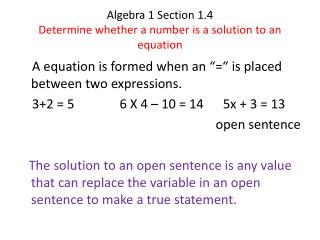DownloadDownload PresentationAlgebra 1 Section 1.4 Determine whether a number is a solution to an equation

# Algebra 1 Section 1.4 Determine whether a number is a solution to an equation

Télécharger la présentation## Algebra 1 Section 1.4 Determine whether a number is a solution to an equation

- - - - - - - - - - - - - - - - - - - - - - - - - - - E N D - - - - - - - - - - - - - - - - - - - - - - - - - - -
##### Presentation Transcript

1. Algebra 1 Section 1.4Determine whether a number is a solution to an equation A equation is formed when an “=“ is placed between two expressions. 3+2 = 5 6 X 4 – 10 = 14 5x + 3 = 13 open sentence The solution to an open sentence is any value that can replace the variable in an open sentence to make a true statement.

2. examples Are x = 5 and x= 3 solutions of 2x + 7 = 17? Are 2, 3, and 4 solutions of x2– 5x = -6 ? Is 3 solution of 4h – h = 12 ÷ h ?

3. Every equation can be expressed verbally. Express verbally. x + 4 = 9 2x – 5 = 17 Activity: page 25

4. Inequality signs < less than < less or equal > greater than > greater or equal Is 6 a solution of 2x + 3 > 9? Is 4 a solution of 5x – 6 < 14?

5. Assignment Page 27 Problems 14 – 64 even, 69 – 76 all, 88,93